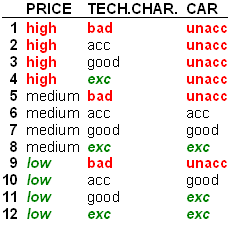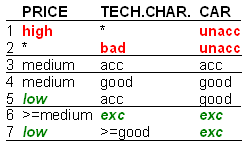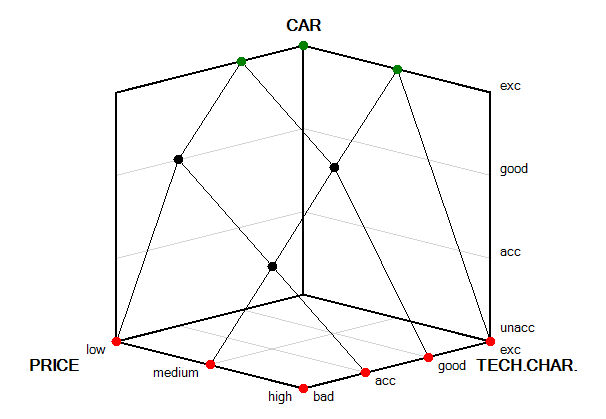# Representing Aggregation Functions

Aggregation functions are sometimes defined with large decision tables that contain 20, 30 or more decision rules. In such cases, it is important to represent such functions in a more compact and comprehensible way. DEX software uses two such representations: using complex rules and three-dimensional (3D) graphics.

## Complex Rules

A more compact representation of a decision table is obtained by joining several elementary rules that have the same function value. In other words, a complex rule represents one or more elementary rules. A complex rule is characterized by using intervals in the conditional part of the rule. The underlying rule-creation algorithm belongs to the class of rule learning algorithms.

The following is the CAR aggregation function from the Car Evaluation model, represented with elementary rules:This is the same function represented with complex rules:Notice that the number of rows has decreased from 12 to 7 and that symbols ‘*’ and ‘>=’ are used in the conditional part of the second table. For example, the complex rule 1 says that if PRICE is ‘high’, and regardless on the value of TECH.CHAR. (denoted ‘*’), the value of CAR is ‘unacc’. This complex rule is a compact representation of the first four elementary rules.

## 3D Graphics

Another way of representing an aggregation function is using 3D graphics. The same CAR function as above is displayed as follows.Functions that have more than two input arguments (such as PRICE and TECH.CHAR. above) are displayed as a series of 3D intersections.